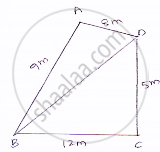# A Park, in the Shape of a Quadrilateral Abcd, Has ∠C = 900, Ab = 9 M, Bc = 12 M, Cd = 5 M and Ad = 8 M How Much Area Does It Occupy? - Mathematics

A park, in the shape of a quadrilateral ABCD, has ∠C = 900, AB = 9 m, BC = 12 m, CD = 5 m and AD = 8 m How much area does it occupy?

#### Solution

Given sides of a quadrilaterals are AB = 9, BC = 12, CD = 05, DA = 08
Let us joint BD
In ΔBCD applying Pythagoras theorem.

BD^2=BC^2+CD^2

=(12)^2+(5)^2
=144+25
=169
𝐵𝐷=13𝑚Area of ΔBCD = 1/2×𝐵𝐶×𝐶𝐷=[1/2×12×5]m^2=30m^2

or ΔABD

S=sqrt(perimeter)/2=sqrt(9+8+13)/2=15cm

By heron’s formulasqrt(s(s-a)(s-b)(s-c))

Area of the triangle =sqrt(15(15-9)(15-8)(15-13))m^2

=35.496 + 30 m^2

= 65.5 m^2 (approximately)

Concept: Application of Heron’s Formula in Finding Areas of Quadrilaterals
Is there an error in this question or solution?

#### APPEARS IN

RD Sharma Mathematics for Class 9
Chapter 17 Heron’s Formula
Exercise 17.2 | Q 4 | Page 19

Share